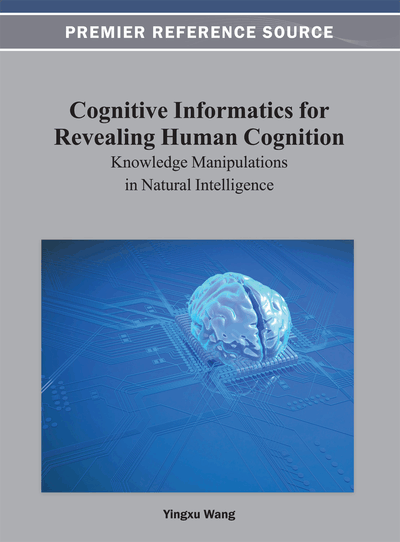# In Search of Effective Granulization with DTRS for Ternary Classification

Bing Zhou (University of Regina, Canada) and Yiyu Yao (University of Regina, Canada)
DOI: 10.4018/978-1-4666-2476-4.ch015

## Abstract

Decision-Theoretic Rough Set (DTRS) model provides a three-way decision approach to classification problems, which allows a classifier to make a deferment decision on suspicious examples, rather than being forced to make an immediate determination. The deferred cases must be reexamined by collecting further information. Although the formulation of DTRS is intuitively appealing, a fundamental question that remains is how to determine the class of the deferment examples. In this paper, the authors introduce an adaptive learning method that automatically deals with the deferred examples by searching for effective granulization. A decision tree is constructed for classification. At each level, the authors sequentially choose the attributes that provide the most effective granulization. A subtree is added recursively if the conditional probability lies in between of the two given thresholds. A branch reaches its leaf node when the conditional probability is above or equal to the first threshold, or is below or equal to the second threshold, or the granule meets certain conditions. This learning process is illustrated by an example.
Chapter Preview
Top

## Brief Introduction To Decision-Theoretic Rough Set Model

Bayesian decision theory is a fundamental statistical approach that makes decisions under uncertainty based on probabilities and costs associated with decisions. Following the discussions given in the book by Duda and Hart (1973), the decision theoretic rough set model is a straightforward application of the Bayesian decision theory.

## Complete Chapter List

Search this Book:
Reset